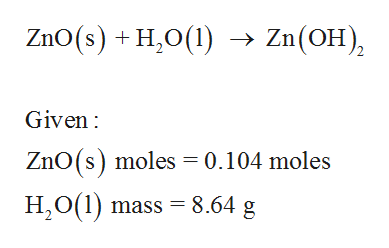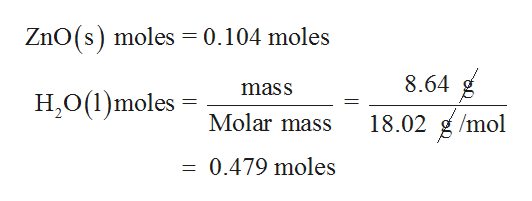# For the following reaction, 0.104 moles of zinc oxide are mixed with 8.64 grams of water.zinc oxide (s) + water (l) zinc hydroxide (aq)What is the FORMULA for the limiting reagent?    What is the maximum amount of zinc hydroxide that can be formed? moles

Question
14 views

For the following reaction, 0.104 moles of zinc oxide are mixed with 8.64 grams of water.

zinc oxide (s) + water (l) zinc hydroxide (aq)

What is the FORMULA for the limiting reagent?

What is the maximum amount of zinc hydroxide that can be formed? moles

check_circle

Step 1

The given balanced reaction is as follows,help_outlineImage TranscriptioncloseZnO (s)H,0(1) Zn(OH) -> Given ZnO (s) moles 0.104 moles H2O(1) mass 8.64 g fullscreen
Step 2

The moles of water present ...help_outlineImage TranscriptioncloseZnO(s) moles = 0.104 moles 8.64 mass H,0(1)moles 18.02 g/mol Molar mass 0.479 moles fullscreen

### Want to see the full answer?

See Solution

#### Want to see this answer and more?

Solutions are written by subject experts who are available 24/7. Questions are typically answered within 1 hour.*

See Solution
*Response times may vary by subject and question.
Tagged in

### General Chemistry